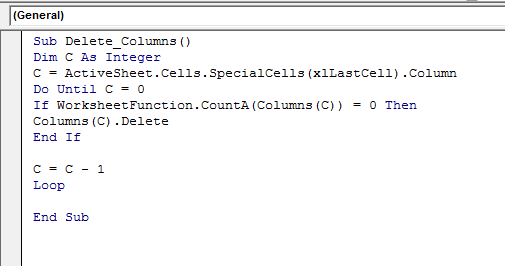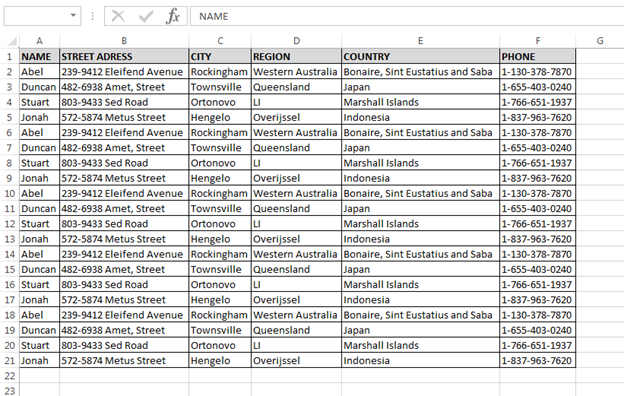How to Delete Empty Columns through VBA in Microsoft Excel?

In this article, we will learn about how to delete empty columns through VBA in Microsoft Excel. We will delete the columns which are completely empty, it means if there is no data within the entire column that data.

Let’s take an example and understand:

We have data in range A1:I21, in which column A contains Name, column B is blank, column C contains Street address, column D City, column E is blank, column F contains region, column G is blank, column H contains country name, and column I contains phone number.We want to delete the blank columns from the data, follow below given steps and code:

• Open the VBE page to press the key Alt+F11
• Insert the module to write the code
• Write the below mentioned code:

```Sub Delete_Columns()

Dim C As Integer

C = ActiveSheet.Cells.SpecialCells(xlLastCell).Column

Do Until C = 0

If WorksheetFunction.CountA(Columns(C)) = 0 Then

Columns(C).Delete

End If

C = C - 1

Loop

End Sub
```

Code Explanation:

First we have defined all the variables. Then the range of blank columns, then we have used “Do until loop” to delete the blank columns. And, now we have defined the blank column and delete command.To run the code press the key F5 on your keyboard,

• All blank columns will get delete.In this way, we can delete the empty columns through VBA in Microsoft Excel.